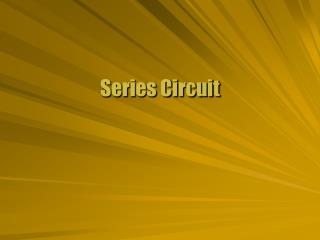DownloadDownload PresentationSeries Circuit

Series Circuit

Télécharger la présentationSeries Circuit

- - - - - - - - - - - - - - - - - - - - - - - - - - - E N D - - - - - - - - - - - - - - - - - - - - - - - - - - -
Presentation Transcript

1. Series Circuit

2. Two circuit elements joined together end to start are in series. One wire connection Electrons don’t pile up in an element as current flows. The two elements have the same current. May have different voltage Same Current

3. Batteries can be joined in series. Joined plus to minus Connecting lines are conductors Total potential is the sum of individual potentials. Normal battery symbol suggests series Series Batteries V1 V2 V3 V

4. Resistors can be joined in series. End doesn’t matter Ohm’s law gives the voltage drop for each resistor. Sum for total Divide by current Total resistance is the sum of individual resistances. Series Resistors R1 R2 R3 I

5. Real voltage sources have some internal resistance. Resistor in series with battery The resistor reduces some voltage from the battery. Same current through internal resistance Ohm’s law for voltage drop Internal Resistance Rint Veff Vint I

6. Power in a circuit is measured in watts (W). Joule/sec A watt is a volt times an amp. Voltage times current Ohm’s law can be combined with the power formula. Eliminate voltage or current Power

7. Flashlight • A flashlight uses a series circuit. • Equivalent batteries and resistances

8. The flashlight uses two 1.5 V batteries with 10 W internal resistance each. The bulb has a resistance of 50 W. Find the current through the flashlight, and the power dissipated by the bulb. The elements form a series circuit. Total EMF of V = 3.0 V Total resistance of R = 70 W Ohm’s law gives the current. I = V/R = 3.0 V / 70 W I = 0.043 A = 43 mA The power is dissipated through the resistors. P = V2 / R = 130 mW Lights On

9. A circuit with a complete loop is a closed circuit. All elements in series Interrupted circuit is open An ammeter measures current and must be in series. Schematic symbol for amps Single Loop Rint Veff Vint I Rint Veff Vint I A

10. Kirchhoff’s Voltage Law • An electron moving in a circuit loop has some potential increases and some decreases. • Increases from batteries, decreases from resistors • The work done by the circuit on an electron in a closed loop must be zero. • Sum of potential changes must be zero • Conservation of energy • This is Kirchhoff’s voltage law. next